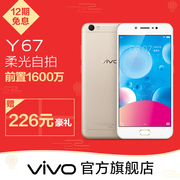# vivo X9前置双摄 全网通4G智能手机 超薄指纹解锁正品分期 vivox9

¥2798.00
58人评价

1. 本平台不售卖商品，请点击直达链接进入vivo官方旗舰店进行购买
• 描述：4.8

• 物流：4.8

• 服务：4.8

• {"list": [{"k":"规格参数","v":[{"k":"拍照功能","v":[{"k":"后置摄像头","v":"1300万像素"},{"k":"摄像头类型","v":"双摄像头（前后）"},{"k":"视频显示格式","v":"1080P(全高清D5)"}]},{"k":"显示","v":[{"k":"屏幕尺寸","v":"5.5英寸"},{"k":"触摸屏类型","v":"电容式"},{"k":"分辨率","v":"1280x720"}]},{"k":"网络","v":[{"k":"网络类型","v":"4G全网通"},{"k":"网络模式","v":"双卡多模"}]},{"k":"机身详情","v":[{"k":"款式","v":"直板"},{"k":"键盘类型","v":"虚拟触屏键盘"},{"k":"厚度","v":"9mm以下"}]},{"k":"存储","v":[{"k":"运行内存RAM","v":"4GB"},{"k":"存储容量","v":"32GB64GB"}]},{"k":"基本参数","v":[{"k":"CPU品牌","v":"联发科"},{"k":"CPU型号","v":"MT6750"},{"k":"品牌","v":"vivo"},{"k":" 型号","v":"y67"},{"k":"上市时间","v":"2016年12月"},{"k":"机身颜色","v":"玫瑰金香槟金磨砂黑星空灰"},{"k":"操作系统","v":"Android/安卓"},{"k":"手机类型","v":"4G手机"},{"k":"电池类型","v":"不可拆卸式电池"},{"k":"核心数","v":"八核"},{"k":"八核CPU频率","v":"1.5G HZ"},{"k":"电池容量","v":"3000mAh"}]}]},{"k":"包装清单","v":[{"k":"主机","v":"1件"},{"k":"耳机","v":"1件"},{"k":"数据线","v":"1件"},{"k":"充电器","v":"1件"},{"k":"透明保护外壳","v":"1件"},{"k":"保修卡","v":"1件"},{"k":"快速入门指南","v":"1件"}]}]}